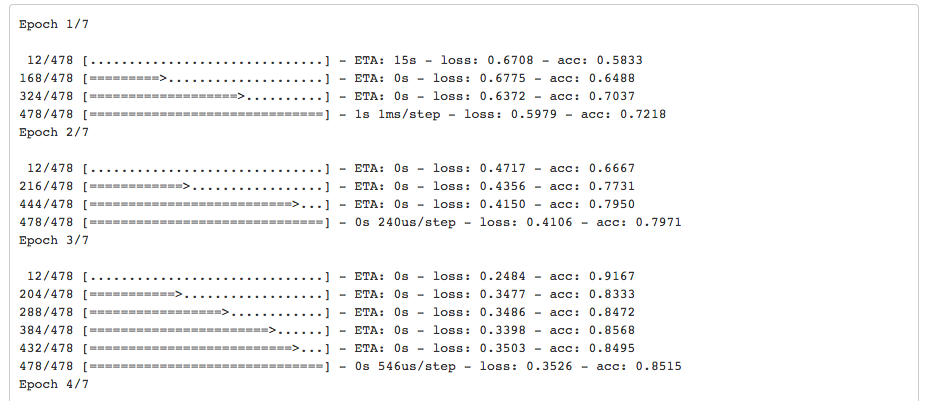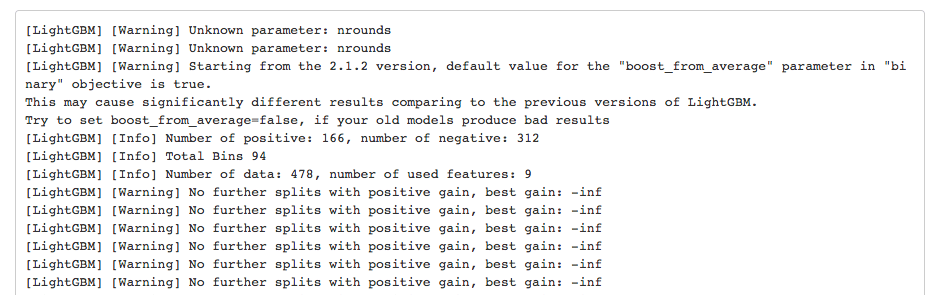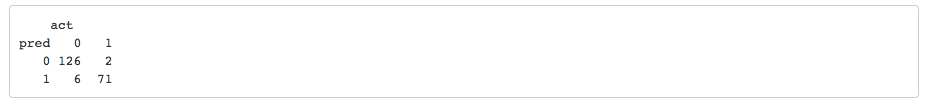# The reticulate package solves the hardest problem in data science: people

March 17, 2019
By

Want to share your content on R-bloggers? click here if you have a blog, or here if you don't.

Andrew Mangano is the Director of eCommerce Analytics at Albertsons Companies.

Part I – Modelling

The `reticulate` package integrates Python within R and, when used with RStudio 1.2, brings the two languages together like never before. Much more important than the technical details of how it all works is the impact that it has on on both individuals and teams by enabling data scientists who speak different languages to collaborate seamlessly on a project.

A data scientist is first and foremost a problem solver. The ability to frame a problem and decide how it might be solved is what separates someone who merely knows code syntax from someone who is capable of discovering a novel solution to a hard problem. Despite all of the buzz around the field, however, there exists a major skills gap where there is limited talent available.

When I meet someone who shares an analytic mindset and a passion for data science, it is exciting. Unfortunately, when it comes to collaboration, the Python/R language gap among practitioners yields an inefficient separation. Python and R did not easily mix previously. Teams would spend valuable analysis time translating and re-coding, or worse, dividing analysts into groups around one language. I recently heard a recruiter say: “if you program in Python, then you should apply to this team and if you program in R, then you should apply to that team.” How absurd it is to throw the problem solver out of the equation and limit team-building to a language!

In this example, I highlight how the `reticulate` package might be used for an integrated analysis. While simple, it highlights three different types of models: native R (`xgboost`), ‘native’ R with Python backend (`TensorFlow`), and a native Python model (`lightgbm`) run in-line with R code, in which data is passed seamlessly to and from Python.

In order to provide an open-source reproducible example, we’ll use the `BreastCancer` data set from the `mlbench` package. Our task is binary classification to predict the class as ‘benign’ or ‘malignant.’

``````library(mlbench) #provides the data set
data("BreastCancer")``````

Many machine learning models require the data frame to be represented as a numeric matrix. Using `sapply()`, we convert the data frame to a numeric matrix. To make the example simpler, we remove incomplete observations via complete.cases and remove the Id column. Converting the ‘Class’ column to numeric creates a numeric column as 1 and 2 instead of 0 and 1, which much be corrected.

The matrix is now ready for a 70% / 30% split for training and testing data sets. The initial training and testing sets are in their native dimensions. One of the model frameworks that we plan to use requires scaled data, which is achieved by using the mean and standard deviation in the scale function.

``````#convert to numeric for models and remove na values for this example
model_set <- sapply(BreastCancer[complete.cases(BreastCancer),-1], as.numeric)

#format target variable as 0, 1 instead of 1,2
model_set[,10]<-model_set[,10]-1

#Split into test and train sets
indices <- sample(1:nrow(model_set), size = 0.7 * nrow(model_set))

#Target variables
target<-unlist(model_set[indices,10])
test_target<-unlist(model_set[-indices,10])

#create unscaled data set for boosted tree models
unscale_train<-as.matrix(model_set[indices,-10])
unscale_test<-as.matrix(model_set[-indices,-10 ])

#create normalized data set for neural network
mean <- apply(model_set[indices,-10], 2, mean)
std <- apply(model_set[indices,-10], 2, sd)

train <- scale(model_set[indices,-10], center = mean, scale = std)
test <- scale(model_set[-indices,-10], center = mean, scale = std)``````

Now that we have a training and testing data set, we can train models.

The first model is a native R package, `xgboost`, short for ‘extreme gradient boosting’. This library can be installed via a simple call of `install.packages('xgboost')`, and does not require any additional software. The objective function for our classification problem is ‘binary:logistic’, and the evaluation metric is ‘auc’ for ‘area under the curve’ in an ROC framework.

``````library(xgboost)

boost_model<-xgboost(data = unscale_train,label=target,booster="gbtree", nfold = 2,nrounds = 25, verbose = FALSE, objective = "binary:logistic", eval_metric = "auc", nthread = 4)``````

The next model is a “native” R Package, `TensorFlow` in R using Keras. Keras is a common interface for TensorFlow, which makes it easier to build certain models. Unlike the previous package, there are extra installation steps for this package beyond `install.packages('keras')`. Once the library is installed, another step is required via `install_keras()`. TensorFlow in R uses a python backend, which is why additional set up is needed. Despite the underlying technical details about how the code works, most users will likely not even notice because the coding is done entirely in R.

The structure and details of this example model are similar to the MNIST example on the RStudio Keras page.

``````library(keras)

y_target<-to_categorical(target,2)

tf_nn <- keras_model_sequential() %>%
layer_dense(units = 12,
activation = 'relu',
input_shape = dim(train)[]) %>%
layer_dropout(rate = 0.4) %>%
layer_dense(units = 12,
activation = 'relu')%>%
layer_dropout(rate = 0.3) %>%
layer_dense(units = 2,
activation = 'softmax')

tf_nn %>% compile(
optimizer = optimizer_rmsprop(),
loss = "categorical_crossentropy",
metrics = c("accuracy")
)

history<-tf_nn %>% fit(
x=train,
y=y_target,
epochs = 7,
batch_size = 12
)``````

(Note that the output has been truncated for publication.)The last model to be tested is entirely outside of the R ecosystem. The goal is to take the data we have been using in R, pass it to python, train a model, then pass the results back into R.

As an example, we will use the Python `LightGBM` package.

To begin, load the reticulate package.

``library(reticulate)``

The next code chunk is written entirely in python. RStudio 1.2 allows chunks of python code to be run in the same notebook as R code. Notice that the beginning of the chunk is not `{r}`, but instead `{python}`.

Data is passed to Python through `r.` commands. In this code chunk, the model turning parameters are saved in `params` and passed in the `lgb.train` function. The data from R is passed in the `r.unscale_train`, `r.target`, `r.unscale_test`. This is the same data used in the `xgboost` model.

``````import pandas as pd
import lightgbm as lgb
params = {
'boosting_type': 'rf',#or can use 'gbdt',#'dart',
'objective': 'binary',
'metric': {'auc'},
'num_leaves': 10,
'max_depth': 8,
'feature_fraction': 0.9,
'bagging_fraction': 0.95,
'bagging_freq': 10,
'learning_rate': 0.003,
'nrounds':10,
'min_data': 10
}

lgtrain = lgb.Dataset(r.unscale_train, label=r.target)
model = lgb.train(params, lgtrain, 200)
light_gbm_test = model.predict(r.unscale_test)``````

(Note that the output has been truncated for publication.)Once the model is trained in Python, it is possible to pass the data back to R using the `py\$` command. In this chunk, which is back in R code, the test set predictions are passed to a data frame in R to compare the performance against the other models.

``lgb_pred<-py\$light_gbm_test``

This last code chunk creates probability and binary predictions for the `xgboost` and `TensorFlow` (neural net) models, and creates a binary prediction for the `lightGBM` model. Using the binary predictions, we then create basic confusion matrices to compare the model predictions on the test data set.

``````xgbpred <- predict (boost_model,unscale_test)
xgbpred_binary <- ifelse (xgbpred > 0.5,1,0)

nn_pred <- tf_nn %>% predict_classes(x=test)
nnpred_prob <- tf_nn %>% predict(x=test)

lgb_pred_binary <- ifelse (lgb_pred > 0.5,1,0)

#XG Boost - Natvie R
data.frame(pred=xgbpred_binary,act=test_target)%>%table()````````````#TensorFlow - 'Native' R
data.frame(pred=nn_pred,act=test_target)%>%table()````````````#LightGBM - Python
data.frame(pred=lgb_pred_binary,act=test_target)%>%table()``````In the first part of this example, I showed how R and Python can be used together in a single notebook for a classification problem. The simplicity with which data can be passed allows for streamlined integration between the two languages.

In Part II, I will show visualization features of the `reticulate` package and RStudio 1.2.

Python version 3.7 – https://www.anaconda.com/

Keras TensorFlow for R – https://tensorflow.rstudio.com/keras/

R-bloggers.com offers daily e-mail updates about R news and tutorials about learning R and many other topics. Click here if you're looking to post or find an R/data-science job.
Want to share your content on R-bloggers? click here if you have a blog, or here if you don't.